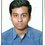# Please Help

If p is prime number and a and b are natural numbers such that a>b, Prove that

$\large{\dbinom{pa}{pb} \equiv \dbinom{a}{b} \mod p}$Note by Md Zuhair
3 years, 8 months ago

This discussion board is a place to discuss our Daily Challenges and the math and science related to those challenges. Explanations are more than just a solution — they should explain the steps and thinking strategies that you used to obtain the solution. Comments should further the discussion of math and science.

When posting on Brilliant:

• Use the emojis to react to an explanation, whether you're congratulating a job well done , or just really confused .
• Ask specific questions about the challenge or the steps in somebody's explanation. Well-posed questions can add a lot to the discussion, but posting "I don't understand!" doesn't help anyone.
• Try to contribute something new to the discussion, whether it is an extension, generalization or other idea related to the challenge.
• Stay on topic — we're all here to learn more about math and science, not to hear about your favorite get-rich-quick scheme or current world events.

MarkdownAppears as
*italics* or _italics_ italics
**bold** or __bold__ bold
- bulleted- list
• bulleted
• list
1. numbered2. list
1. numbered
2. list
Note: you must add a full line of space before and after lists for them to show up correctly
paragraph 1paragraph 2

paragraph 1

paragraph 2

[example link](https://brilliant.org)example link
> This is a quote
This is a quote
    # I indented these lines
# 4 spaces, and now they show
# up as a code block.

print "hello world"
# I indented these lines
# 4 spaces, and now they show
# up as a code block.

print "hello world"
MathAppears as
Remember to wrap math in $$ ... $$ or $ ... $ to ensure proper formatting.
2 \times 3 $2 \times 3$
2^{34} $2^{34}$
a_{i-1} $a_{i-1}$
\frac{2}{3} $\frac{2}{3}$
\sqrt{2} $\sqrt{2}$
\sum_{i=1}^3 $\sum_{i=1}^3$
\sin \theta $\sin \theta$
\boxed{123} $\boxed{123}$

## Comments

Sort by:

Top Newest

Well, $\binom{pa}{pb} = \frac{(pa)!}{(pb)!(pa-pb)!},$ and now look at the terms on the top and bottom that are divisible by $p.$ These are $\frac{(pa)(p(a-1))(p(a-2))(\cdots)(p\cdot 1)}{(pb)(p(b-1))(\cdots)(p\cdot 1)(p(a-b))(p(a-b-1))(\cdots)(p\cdot 1)} = \frac{p^a a!}{p^b b! \cdot p^{a-b} (a-b)!} = \frac{a!}{b!(a-b)!} = \binom{a}{b}.$ What are the leftover terms mod $p$? It's just a bunch of copies of $(p-1)!$: $\frac{(p-1)!^a}{(p-1)!^b(p-1)!^{a-b}} \equiv 1.$ So that'll do it.

You might be interested in the Lucas' theorem wiki.

- 3 years, 8 months ago

Log in to reply

Can anyone help in this one?

- 3 years, 8 months ago

Log in to reply

This is a lemma in the proof of lucas' theorem.

In fact, there is a stronger statement.

For primes $p \geq 5$, ${ pa \choose pb } \equiv { a \choose b } \pmod{p^3}$.

Staff - 3 years, 8 months ago

Log in to reply

Sir how do we find the range of p for $\large{{ pa \choose pb} \equiv {a \choose b} \mod p^4}$ ?

- 3 years, 8 months ago

Log in to reply

I doubt that holds for a large range.

The $p^3$ case is also known as wolstenholme's theorem, and the ideas have been used in several olympiad problems.

Staff - 3 years, 8 months ago

Log in to reply

For the need of Olympiads only i am learning this.. RMO is this Sunday. :)

Wish a best of luck for me.

You were one of those people who represented the your motherland. Proud of you :)

- 3 years, 8 months ago

Log in to reply

@Md Zuhair @Rahil Sehgal How may questions did you solve in RMO 2017 ???

- 3 years, 8 months ago

Log in to reply

U first... hw many?

- 3 years, 8 months ago

Log in to reply

@Md Zuhair I solved 4, but I didnt get the time to write 1 question completely....so effectively I did 3.5... What abt you??

- 3 years, 8 months ago

Log in to reply

Did 3. But unsure

- 3 years, 8 months ago

Log in to reply

Which ones?

- 3 years, 8 months ago

Log in to reply

Inequality, Number theory and Combinatorics

- 3 years, 8 months ago

Log in to reply

×

Problem Loading...

Note Loading...

Set Loading...